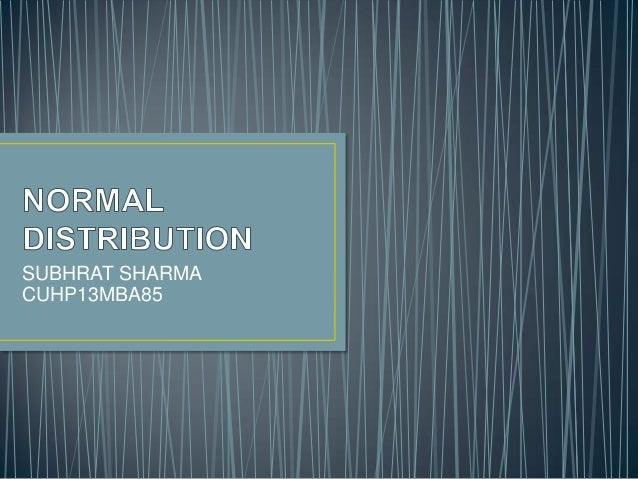Successfully reported this slideshow.
We use your LinkedIn profile and activity data to personalize ads and to show you more relevant ads. You can change your ad preferences anytime.Upcoming SlideShare
Loading in …5
×

# Normal distribution

it is a ppt with just properties of normal curves. n few of other things about normal distribution.

• Full Name
Comment goes here.

Are you sure you want to Yes No
Your message goes here• Login to see the comments

### Normal distribution

1. 1. SUBHRAT SHARMA CUHP13MBA85
2. 2. • Each binomial distribution is defined by n, the number of trials and p, the probability of success in any one trial. • Each Poisson distribution is defined by its mean • In the same way, each Normal distribution is identified by two defining characteristics or parameters: its mean and standard deviation. • The Normal distribution has three distinguishing features: • It is unimodal, in other words there is a single peak. • It is symmetrical, one side is the mirror image of the other. • It is asymptotic, that is, it tails off very gradually on each side but the line representing the distribution never quite meets the horizontal axis
3. 3. • It is symmetric around the point x = μ, which is at the same time the mode, the median and the mean of the distribution. • It is unimodal: its first derivative is positive for x < μ, negative for x > μ, and zero only at x = μ. • It has two inflection points (where the second derivative of f is zero and changes sign), located one standard deviation away from the mean, namely at x = μ − σ and x = μ + σ. • It is log-concave • It is infinitely differentiable, indeed super smooth of order 2
4. 4. Probability density function Cumulative distribution function
5. 5. • When number of trials increase , probability distribution tends to normal distribution .hence , majority of problems and studies can be analysed through normal distribution • Used in statistical quality control for setting quality standards and to define control l